# 1 Description

This vignette demonstrates the multi-group integration framework of MOFA+ on a single data modality.

We consider a data set of scRNA-seq experiments where 16,152 cells were isolated from a total of 8 mouse embryos from developmental stages E6.5, E6.75, E7.0 and E7.25 (two embryos per stage), spanning post-implantation and early gastrulation.
Despite differences in developmental time, embryos are expected to contain similar subpopulations of cells. Hence, MOFA+ should detect the existence of biological sources of variation that are shared between groups.

The data set we use here is a simplified subset version of the original data set, which can be visualised and downloaded from here.

# 2 How does the multi-group inference work?

Intuitively, this extension breaks the assumption of independent samples and allows inference across multiple groups, where groups are predefined sets of samples (i.e. different conditions, batches, cohorts, etc.).
Importantly, the model is not focused on capturing the differential changes between the groups (as for example when doing differential expression). The aim of the multi-group framework is to find out the sources of variability within each group and to charactersie which ones are shared between the different groups from those that are exclusive to a single group.

This is a rather advanced option that we disencourage if this is the first time that you are using MOFA. For more questions, please read the FAQ section.

# 3 Load libraries and data

## 3.1 Libraries

Make sure that `MOFA2` is imported last, to avoid collisions with functions from other packages

``````library(Seurat)
library(ggplot2)
library(MOFA2)``````

## 3.2 Data

The RNA expression data has been processed using Seurat. It has already been normalised and subset to the top 5,000 most variable genes (after regressing out the group effect).

``````load(url("ftp://ftp.ebi.ac.uk/pub/databases/mofa/scrna_gastrulation/gastrulation10x_seurat.RData"))
seurat``````
``````## An object of class Seurat
## 5000 features across 16152 samples within 1 assay
## Active assay: RNA (5000 features, 5000 variable features)``````

Define cell type colors for the visualisations

``````colors <- c(
"Epiblast" = "grey70",
"Primitive Streak" = "sandybrown",
"Mesoderm" = "violetred",
"ExE endoderm" = "#548B54",
"ExE ectoderm" = "black"
)``````

# 4 Create MOFA object

Group cells according to the embryo and the stage they comne from

``````seurat@meta.data\$stage_sample <- paste(seurat@meta.data\$stage,seurat@meta.data\$sample, sep="_")
unique(seurat@meta.data\$stage_sample)``````
``##  "E6.5_1"   "E6.5_5"   "E7.0_10"  "E7.0_14"  "E7.25_26" "E7.25_27"``
``MOFAobject <- create_mofa(seurat, groups = "stage_sample")``

## 4.1 Plot data overview

``plot_data_overview(MOFAobject)``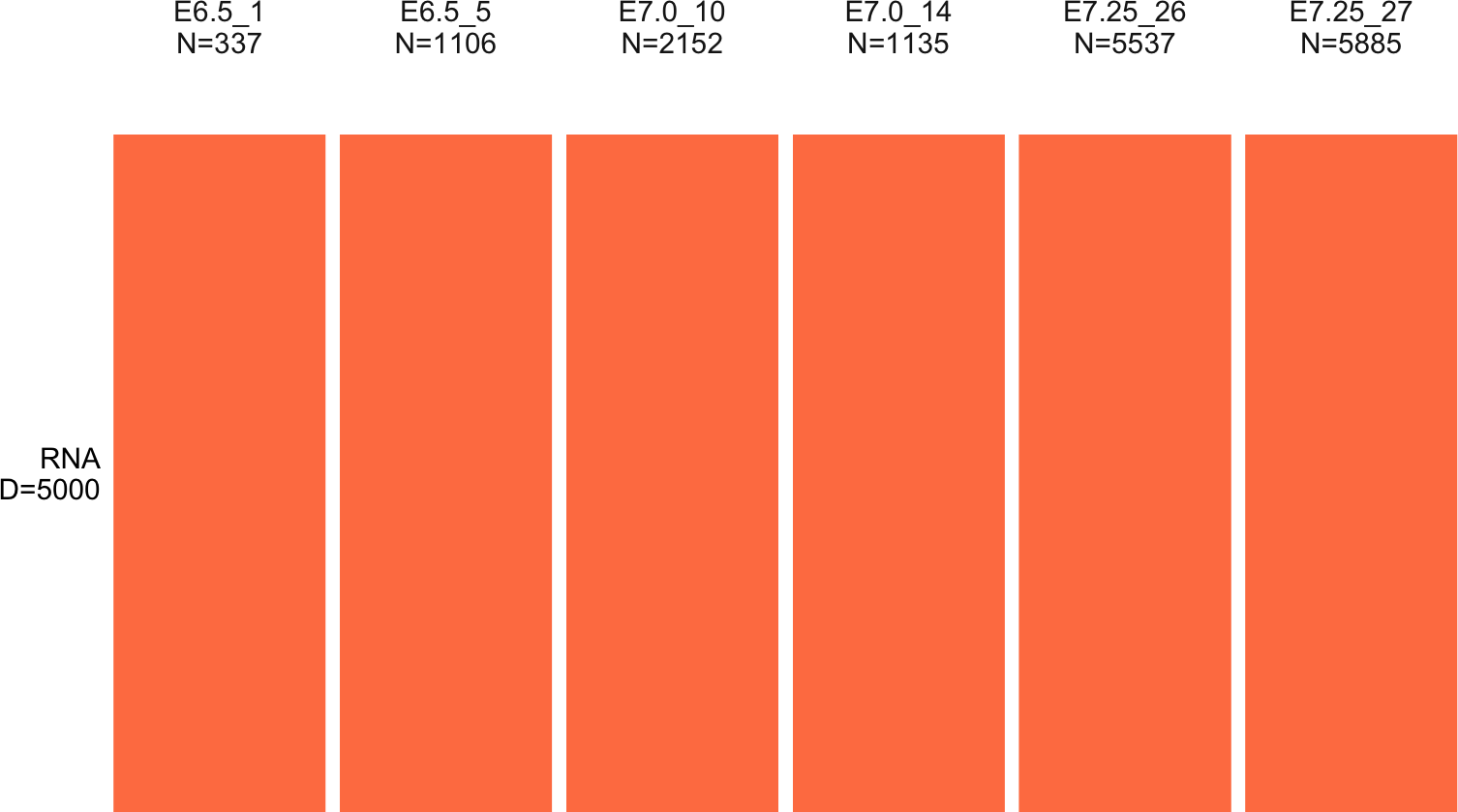## 4.2 Define MOFA options

``````# Default data options
data_opts <- get_default_data_options(MOFAobject)

# Default model options
model_opts <- get_default_model_options(MOFAobject)

# Training options
train_opts <- get_default_training_options(MOFAobject)
train_opts\$convergence_mode <- "fast"
train_opts\$seed <- 42``````

## 4.3 Prepare the MOFA object

``````MOFAobject <- prepare_mofa(
object = MOFAobject,
data_options = data_opts,
model_options = model_opts,
training_options = train_opts
)``````

# 5 Train the MOFA model

This step can take quite some time (~1h with standard CPU inference), so we provide a pre-trained model in the next chunk of code.

Note: if you train the model from scratch, the results will not be 100% reproducible with the pre-trained model (but hopefully similar enough!).

``MOFAobject <- run_mofa(MOFAobject)``

MOFA models are saved in hdf5 format and can be loaded into R with the function `load_model`. In this case, however, we provide the trained model as an `RData` file

``````# MOFAobject <- load_model(outfile)

# 6 Overview of the trained MOFA model

The MOFAobject consists of multiple slots where relevant data and information is stored. For descriptions, you can read the documentation by `?MOFA`

``slotNames(MOFAobject)``
``````##   "data"               "intercepts"         "imputed_data"
##   "training_stats"     "training_options"   "stochastic_options"
##  "data_options"       "model_options"      "dimensions"
##  "on_disk"            "dim_red"            "cache"
##  "status"``````

`dimensions` contains the dimensions of the model (K for factors, M for views, G for groups, N for samples (per group), D for features (per view)):

``MOFAobject@dimensions``
``````## \$M
##  1
##
## \$G
##  6
##
## \$N
##  E6.5 (1)  E6.5 (2)  E7.0 (1)  E7.0 (2) E7.25 (1) E7.25 (2)
##       337      1106      2152      1135      5537      5885
##
## \$D
##  RNA
## 5000
##
## \$K
##  10``````

`data` contains the centered (and optionally scaled) input data matrices. It is a nested list of matrices where the first index refers to the view, and the second index to the group. In this case we have one view (RNA) and six groups

``names(MOFAobject@data)``
``##  "RNA"``
``names(MOFAobject@data[])``
``##  "E6.5 (1)"  "E6.5 (2)"  "E7.0 (1)"  "E7.0 (2)"  "E7.25 (1)" "E7.25 (2)"``

`samples_metadata` contains the cell metadata that I have previously added to the MOFA object. Columns are:

• stage: developmental stage.
• lineage: cell type annotation.
• group: the grouping used for MOFA. In this case each embryo corresponds to a separate group.
``head(MOFAobject@samples_metadata)``
``````##   sample stage          lineage    group
## 1 cell_1  E6.5         Epiblast E6.5 (1)
## 2 cell_2  E6.5 Primitive Streak E6.5 (1)
## 3 cell_5  E6.5     ExE ectoderm E6.5 (1)
## 4 cell_6  E6.5         Epiblast E6.5 (1)
## 5 cell_8  E6.5         Epiblast E6.5 (1)
## 6 cell_9  E6.5         Epiblast E6.5 (1)``````

## 6.1 Overview of training data

The function `plot_data_overview` can be used to obtain an overview of the input data. It shows how many views (rows) and how many groups (columns) exist, what are their corresponding dimensionalities and how many missing information they have (grey bars).
In this case we have one view (RNA expression, a total of 5,000 genes) and 6 groups that correspond to different embryos at different stages of development, for a total of 16,152 cells.

``plot_data_overview(MOFAobject, colors = c("RNA"="darkgreen"))``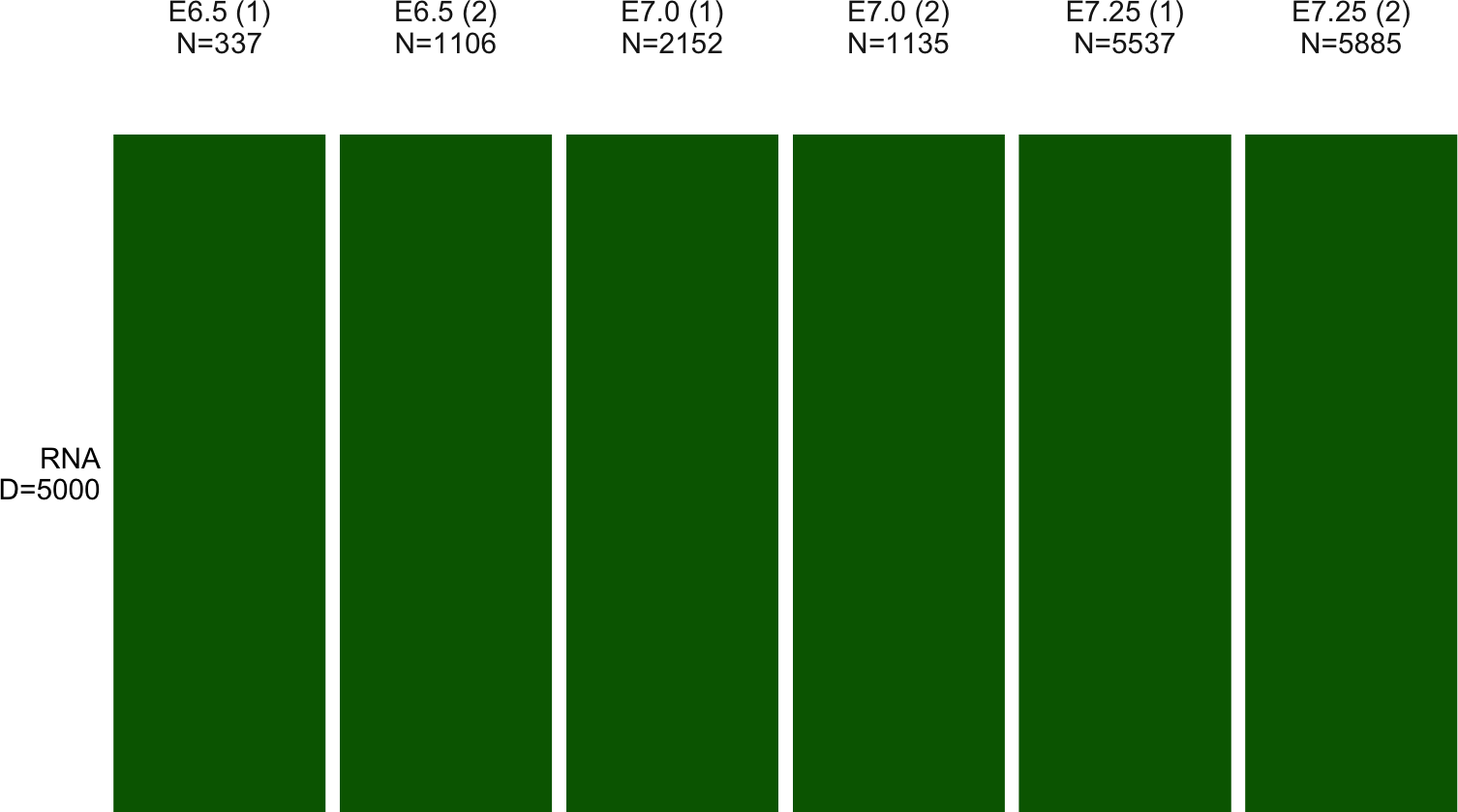# 7 Plot variance explained

Quantifying the variance explained per factor across groups and views is probably the most important plot that MOFA+ generates. It summarises the (latent) signal from a complex heterogeneous data set in a single figure.

## 7.1 Plot variance explained per factor across groups

``plot_variance_explained(MOFAobject, x="group", y="factor")``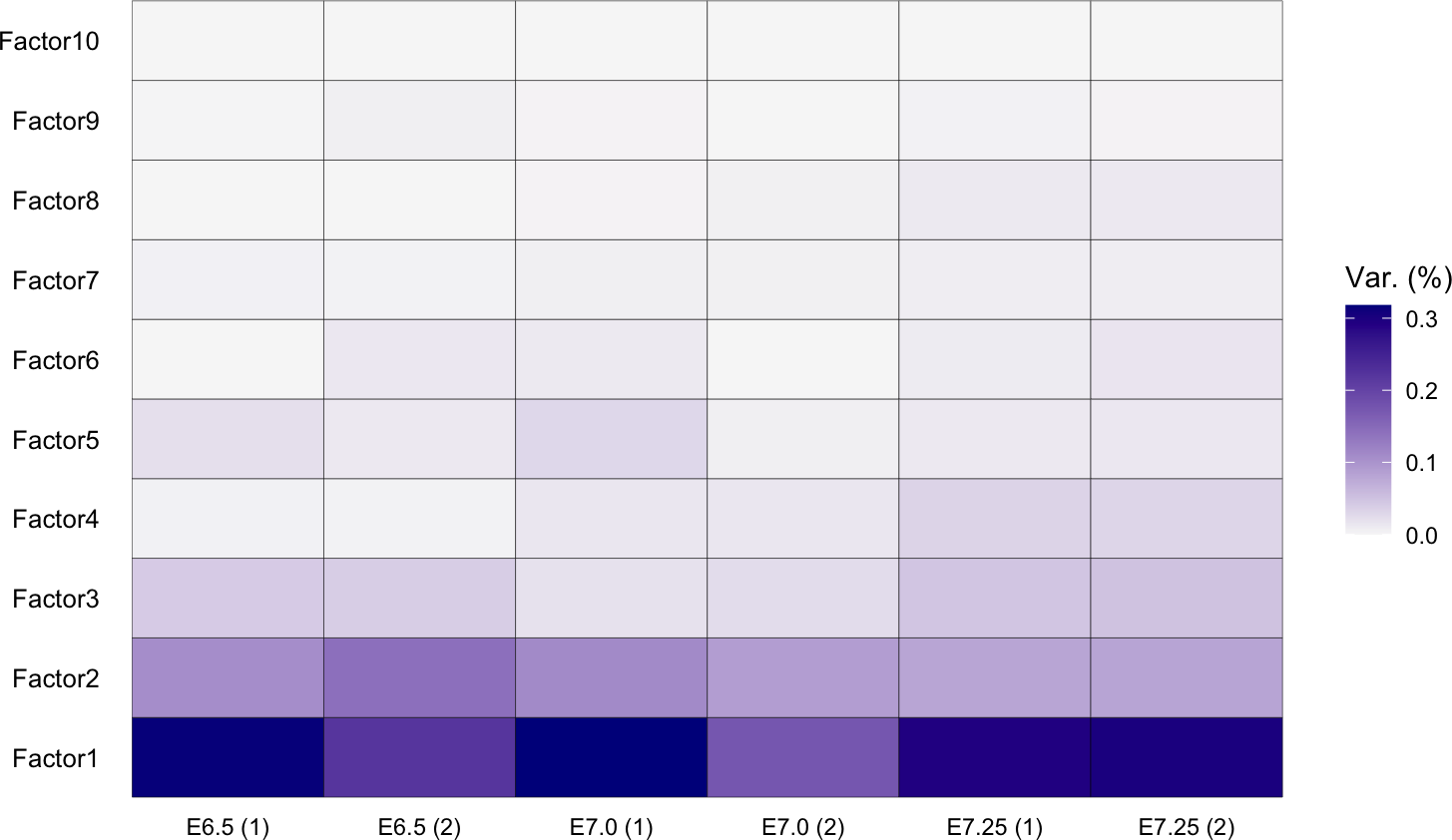There is a lot of information contained in this plot. Factor 1 and Factor 2 explain a lot of variance across multiple groups. In contrast, Factor 4 increases its activity from E6.5 to E7.5, indicating that it captures a source of variation that emerges at E6.5.

We can also plot the total variance explained per group (with all factors) by adding the argument `plot_total = TRUE`. Notably, only 10 factors are sufficient to capture between 35% and 55% of the transcriptional variance per embryo

## 7.2 Plot total variance explained per group

``````plot_variance_explained(
MOFAobject,
x = "group",
y = "factor",
plot_total = T
)[]``````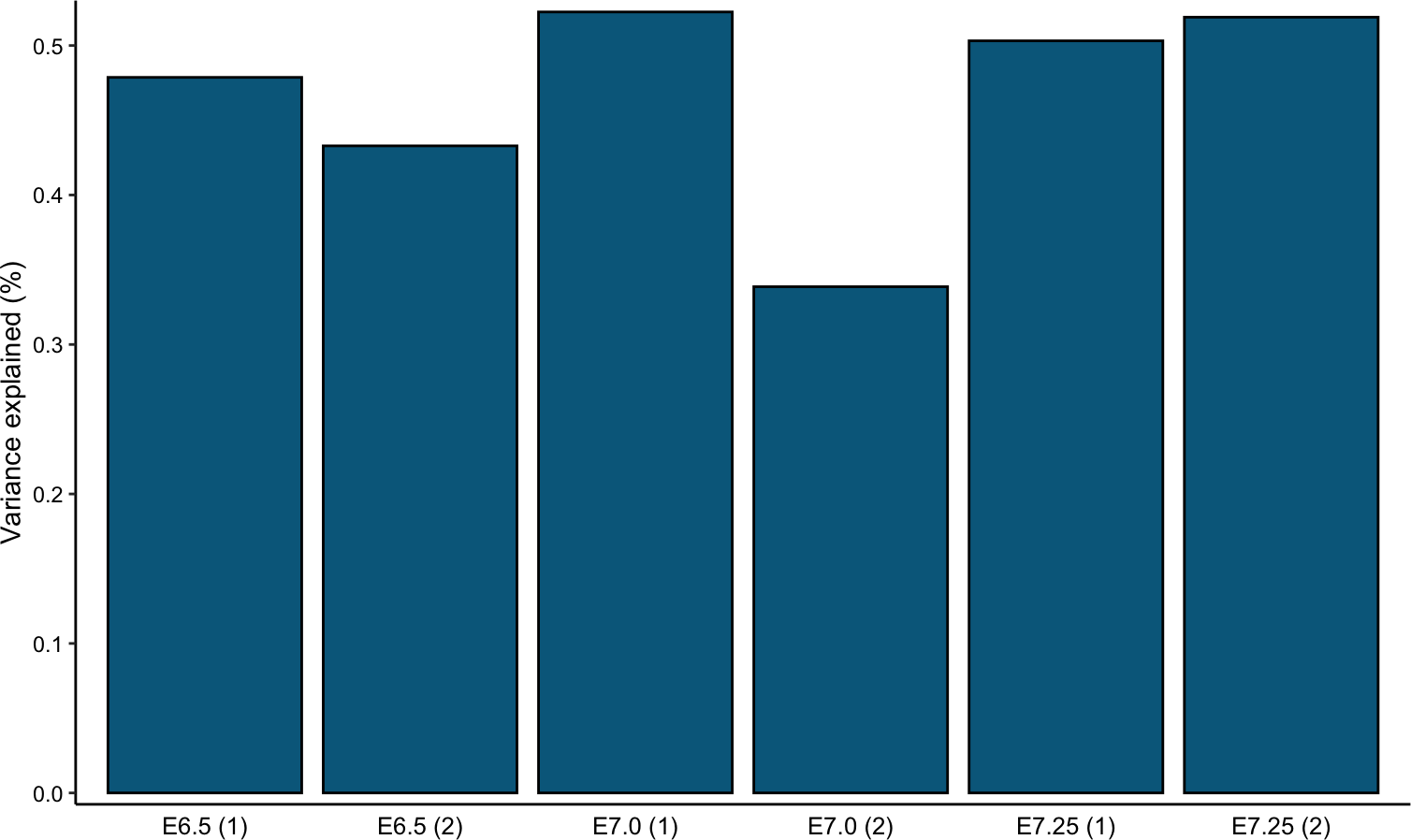## 7.3 Plot variance explained for individual features

We can also inspect the variance explained by the MOFA factors for individual features. A high \(R^2\) implies that the MOFA factors captures most of the variation for this feature, whereas small values means that the variation for this feature is not explained by the model (i.e. it is considered as noise):

Variance explained by all factors, in each group:

``````features <- c("Rbp4","Ttr","Spink1","Mesp1","E130311K13Rik","Hey1")

plot_variance_explained_per_feature(
MOFAobject,
view = "RNA",
features = features
)``````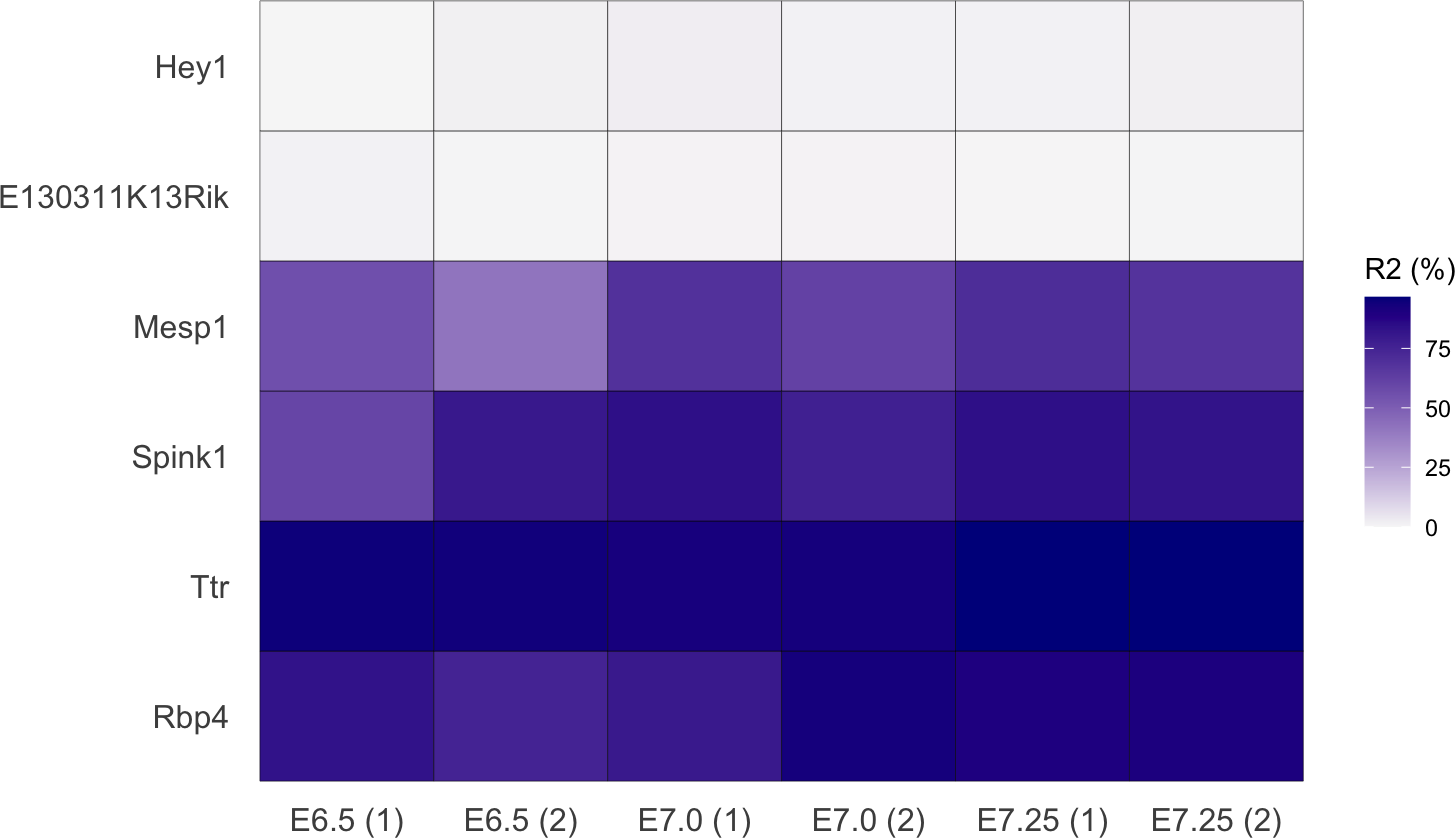# 8 Characterisation of Factor 1

## 8.1 Visualisation of factor values

Each factor ordinates cells along a one-dimensional axis that is centered at zero. Samples with different signs indicate opposite phenotypes, with higher absolute value indicating a stronger phenotype. For example, if the \(k\)-th factor captures the variability associated with cell cycle, we could expect cells in Mitosis to be at one end of the factor (irrespective of the sign, only the relative positioning being of importance). In contrast, cells in G1 phase are expected to be at the other end of the factor. Cells with intermediate phenotype, or with no clear phenotype (i.e. no cell cycle genes profiled), are expected to be located around zero.

Let’s plot Factor 1 values and we color cells by lineage assignment. Clearly, this factors captures the emergence of ExE endoderm.

``````plot_factor(MOFAobject,
factor = 1,
color_by = "lineage"  # lineage is a column in MOFAobject@samples.metadata
) + scale_fill_manual(values=colors)``````